Courses

# Olympiad Test: Lines And Angles

## 20 Questions MCQ Test Mathematics Olympiad Class 7 | Olympiad Test: Lines And Angles

Description
This mock test of Olympiad Test: Lines And Angles for Class 7 helps you for every Class 7 entrance exam. This contains 20 Multiple Choice Questions for Class 7 Olympiad Test: Lines And Angles (mcq) to study with solutions a complete question bank. The solved questions answers in this Olympiad Test: Lines And Angles quiz give you a good mix of easy questions and tough questions. Class 7 students definitely take this Olympiad Test: Lines And Angles exercise for a better result in the exam. You can find other Olympiad Test: Lines And Angles extra questions, long questions & short questions for Class 7 on EduRev as well by searching above.
QUESTION: 1

### What is the supplement of 64°?

Solution:

180° – 64° = 116°

QUESTION: 2

### What is the value of x in the given figure?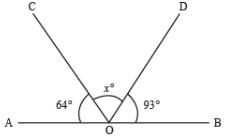Solution:

64 + x + 93 = 180
⇒ 157 + x = 180°
⇒ x = 180° – 157° = 23°

QUESTION: 3

### In the given figure two straight lines AB and PQ intersect at a point O. If ∠AOP = 47° what is the measure of ∠BOQ?

Solution:
QUESTION: 4

What is the complement of 67°?

Solution:
QUESTION: 5

​In the following figure, if AB ∥ CD, ∠ BAP = 108° and ∠ PCD = 120°, what is the measure of ∠ APC?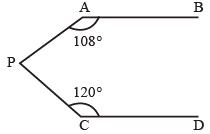Solution: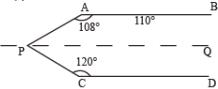AB ∥ PQ
∴ ∠APQ = 180° – 108° = 72°
PQ ∥CD
∠ CPQ = 180° –120° = 60°
∠ APC= ∠APQ+ ∠CPQ = 72° + 60°
= 132°

QUESTION: 6

In the given figure MN ∥ PQ ∠MNE = 120°, ∠EPQ = 100°.What is the value of x?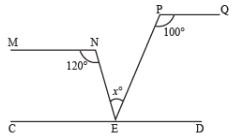Solution: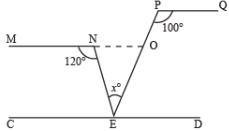Given, MN ∥PQ
∠PON = 100°
∴ ∠NOE = 180° – 100 = 80°
∠ENO = 180° – 120° = 60°
x = 180° – (80° + 60°)
= 180° –140° = 40°

QUESTION: 7

In the given figure AB ∥ CD and EF is transversal. If ∠1 and ∠2 are in the ratio 5:7 what is the measure of ∠8?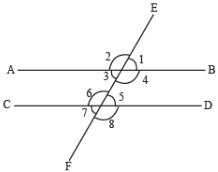Solution:

5x + 7x = 180°
⇒ 12x = 180° ⇒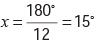∠1 = 5x = 5×15 = 75°
∠2 = 7x = 7×15 = 105°
∠1 = ∠5 = 75°
∠8 = 180° – 75° = 105°

QUESTION: 8

What is the value of x in the following figure?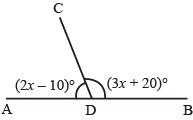Solution:

Here, 2x –10° + 3x + 20° = 180°
⇒ 5x + 10° = 180°
⇒  5x = 170°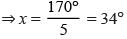QUESTION: 9

Which of the following angle is its complement?

Solution:

x + x = 90° ⇒ 2x = 90° ⇒ x =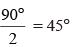QUESTION: 10

In the given figure, ABC is a straight line. What is the value of y?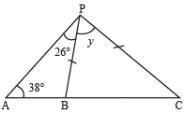Solution: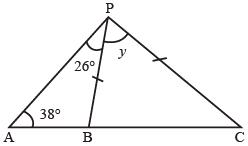In ∆ABP
∠ ABP = 180° –(26°+38°)
= 180° – 64 = 116°
∠PBC = 180° – 116° = 64°
PB = PC
∠PBC = 64°
y = 180° – (64° + 64°)
= 180° –128°
= 52°

QUESTION: 11

In the given figure, PQR is a straight line. What is the value of x?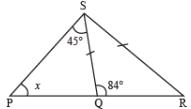Solution:

x + 45° = 84°
x = 84° – 45° = 39°

QUESTION: 12

In the given figure, ABC is a straight line what is the value of z?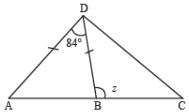Solution: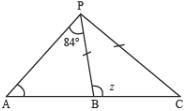In ∆ PAB,
∠PAB+ ∠PBP + 84° = 180°
∠PBA + ∠PBA = 180° – 84°
2 ∠PBA = 96°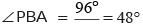z + 48° = 180° ⇒ z = 180° – 48 = 132°

QUESTION: 13

In the given figure, what is the length of BC if AB = AC?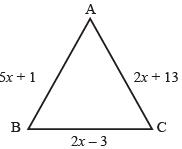Solution:

AB = AC
⇒ 5x + 1 = 2x +13
⇒ 5x –2x = 13 –1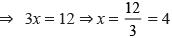and BC = 2x –3 = 2×4 –3 = 5

QUESTION: 14

In the given figure △ACD is a right angled triangle. △ABC is an isosceles triangle with AB = A C. ACQ, E AD, are straight lines. What is the value of x?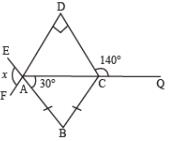Solution:In ∅ACD ∠DAC = 180° – 140° = 40°
∠DAC = 180° – (90° + 40°) = 50°
∠DAB = 50°+30° = 80°
x = ∠DAB = 80°

QUESTION: 15

If the sides of a triangle are as given in the figure. The perimeter of triangle is 78 m. What is the measure of the highest side?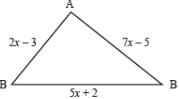Solution:

Perimeter of triangle ABC = 78
∴ 2x –3 + 7x –5 + 5x + 2 = 78
⇒ 14x –6 = 78
⇒ 14x = 78 + 6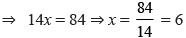Sides are 5x + 2 = 5× 6 + 2 = 32
7x –5= 7× 6 – 5 = 37
2x –3= 2× 6 – 3 = 9

QUESTION: 16

In the given figure △ABC is an equilateral triangle and △ACD is an isosceles triangle.What is the value of x?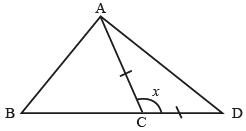Solution:

∠ ABC = ∠ ACB = 60°
At point C,
x + 60° = 180°
⇒ x = 180° – 60° ⇒ x = 120°

QUESTION: 17

If l ∥m, then what is the value of x?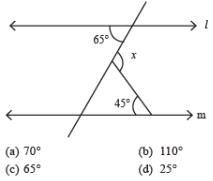Solution: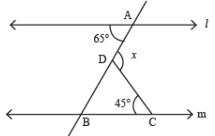∠DBC = 65° as l ∥ m.
∠BDC = 180° –(65° + 45°)
∠BDC = 180° –110° = 70°
x = 180° –70° = 110°

QUESTION: 18

​In the given figure, y/x = 5 and z/x = 4 then what is the value of x?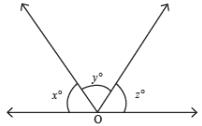Solution: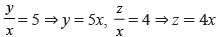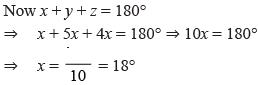QUESTION: 19

Find the value of x in figure given below.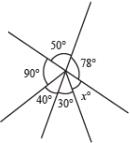Solution:

At point O,
78° + 50° + 90° + 40° + 30° + x = 360°
⇒ 288° + x = 360°
x = 360° –288° = 72°

QUESTION: 20

∠A = 56°, CE ∥ BA ∠ECD = 73°. What is the value of ∠ACB?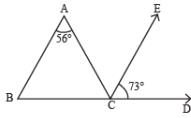Solution:

BA ∥ CE
∠BAC = ∠ACE = 56°
At point C,
⇒ ∠ACB+ ∠ACE+ ∠ECD = 180°
⇒ ∠ACB + 56° + 73° = 180°
⇒ ∠ACB = 180° –(56° + 73°)
⇒ ∠ACB = 180° – 129° = 51°